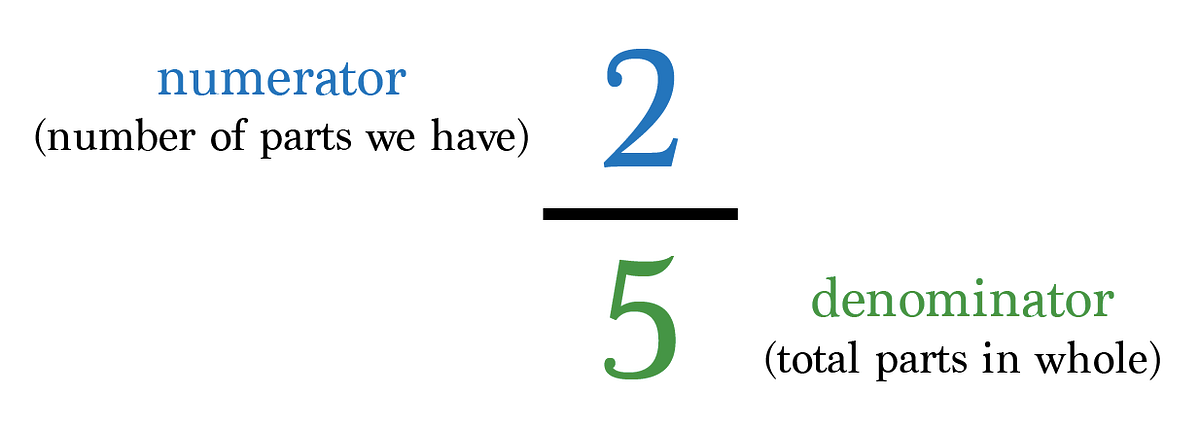# Fractions

Fractions in the Rhythmical Musical Context

Understanding fractions is an important conception to have when consulting music. In music fractions are mainly found in rhythm by way of meters, note division, note subdivision, tempo, and harmonic rhythm. Fractions are what allow musicians to simplify rhythm and help to explain why certain rhythmic patterns sound the way they do.

A fraction is simply defined as a mathematical expression that constitutes the division of a whole number by another whole number. The main uses of fractions consist of describing either a non-whole number (like 1.5, 2.7, or 22.04) or to describe a number whose decimal is infinite (like 5/7 or 9/11).

Fractions are written with the numerator over the denominator. The numerator is the number that is divided by the denominator. In a fraction, the numerator will always appear on top and the denominator will appear below. The fraction essentially forms a small division problem to describe a number.

Here is a diagram of what a typical fraction is notated as:This is because when the numerator (2) is divided by the denominator (5) one will get 0.4.

For example,

When we divide 2 by 5 we end up with 0.4.

2 ÷ 5 = 0.4

To sum it all up, fractions are just small division problems made up of the numerator and denominator separated by a line. This ‘line’ that separates them can be observed as a division symbol or ÷.